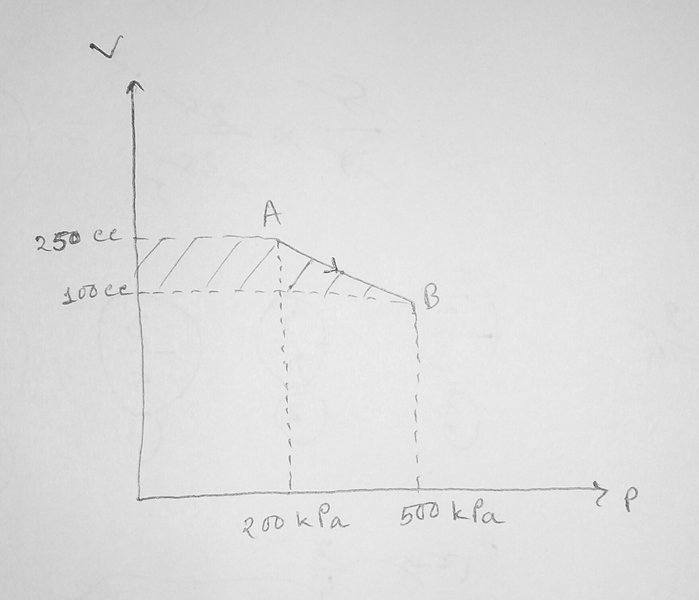# Simple thermodynamics problem<Moderator's note: Approved although without template. Member has been warned.>

An ideal gas is taken along the path AB (see fig.). If 70 calories of heat is extracted from the gas during the process, calculate the change in internal energy during the process.
My attempt :
Work done in the process is the area of the shaded region which turns out to be 52.5 Joules.
Again, heat extracted,
$$Q=-70 \, \text{cal}= -294 \, J$$

So, from first law of thermodynamics,
\begin{align}\Delta U &= Q-W\\ &=(-294-52 5)\, J\\ &= \boxed{-346.5 \, J}\end{align}

So, that was my answer. But my teacher says that the answer is -241.5 Joules. For that, he says that the work done should be taken as negative. What can the possible explanation for this ? Where am I wrong ?

#### Attachments

Last edited by a moderator:

## Answers and Replies

Homework Helper
Gold Member
Energy put into the system is counted positive and vice versa. The heat is extracted, therefore it has a negative sign. So you have to determine whether the work is done by the system or put into the system. The formula could be a help or also just think how work is done on a closed system.

Energy put into the system is counted positive and vice versa. The heat is extracted, therefore it has a negative sign. So you have to determine whether the work is done by the system or put into the system. The formula could be a help or also just think how work is done on a closed system.
Please don't confuse me more. All I need is a proper reasoning which is not required in the homework problem. As you can see, I have got my answer. I can get my teacher's answer easily too by putting a negative sign in front of the work done. So, please explain me in simple words.

Homework Helper
Gold Member
Please don't confuse me more. All I need is a proper reasoning which is not required in the homework problem. As you can see, I have got my answer. I can get my teacher's answer easily too by putting a negative sign in front of the work done. So, please explain me in simple words.

Is the gas compressed or expanded? Plus: The formula would help, I recommend to write it down.